# Planning Methods: Predicting Census Tract change

In this lab, you will use Philadelphia's 2000 Census Tract data to make predictions about the 2010 and 2020 population, and to understand what types of Census Tracts have been growing or shrinking. Download the data here (password is PennDesign). You can load it by double clicking on the file or using the load command.

Start by summarizing the data:

``````summary(dat2000)
``````
``````##     Geo_FIPS          pop_change         pop_plus10        pop
##  Min.   :4.21e+10   Min.   :-1310.00   Min.   :   0   Min.   :    0
##  1st Qu.:4.21e+10   1st Qu.: -394.50   1st Qu.:2721   1st Qu.: 2329
##  Median :4.21e+10   Median :  -85.00   Median :3774   Median : 4008
##  Mean   :4.21e+10   Mean   :  -43.79   Mean   :3919   Mean   : 3983
##  3rd Qu.:4.21e+10   3rd Qu.:  309.50   3rd Qu.:4987   3rd Qu.: 5630
##  Max.   :4.21e+10   Max.   : 2311.00   Max.   :9022   Max.   :10479
##                     NA's   :215        NA's   :106    NA's   :109
##   pop_density     square_miles     pop_white_nonhispanic   pop_black
##  Min.   :    0   Min.   :0.06491   Min.   :   0          Min.   :   0
##  1st Qu.: 8462   1st Qu.:0.18056   1st Qu.: 114          1st Qu.: 166
##  Median :15692   Median :0.25657   Median : 821          Median : 903
##  Mean   :16120   Mean   :0.35457   Mean   :1695          Mean   :1721
##  3rd Qu.:22543   3rd Qu.:0.37694   3rd Qu.:2901          3rd Qu.:2959
##  Max.   :54824   Max.   :3.26893   Max.   :8948          Max.   :8468
##  NA's   :109     NA's   :109       NA's   :109           NA's   :109
##    pop_asian       pop_hispanic      households   households_family
##  Min.   :   0.0   Min.   :   0.0   Min.   :   0   Min.   :   0.0
##  1st Qu.:  13.0   1st Qu.:  33.0   1st Qu.: 852   1st Qu.: 428.0
##  Median :  46.0   Median :  89.0   Median :1502   Median : 899.0
##  Mean   : 177.6   Mean   : 336.7   Mean   :1549   Mean   : 924.8
##  3rd Qu.: 168.0   3rd Qu.: 230.0   3rd Qu.:2187   3rd Qu.:1323.0
##  Max.   :2625.0   Max.   :6802.0   Max.   :6115   Max.   :2738.0
##  NA's   :109      NA's   :109      NA's   :109    NA's   :109
##  households_nonfamily  avg_hh_size     pop_25_plus   edu_less_highschool
##  Min.   :   0         Min.   :0.000   Min.   :   0   Min.   :   0.0
##  1st Qu.: 303         1st Qu.:2.220   1st Qu.:1366   1st Qu.: 286.0
##  Median : 529         Median :2.520   Median :2422   Median : 661.0
##  Mean   : 624         Mean   :2.424   Mean   :2536   Mean   : 729.9
##  3rd Qu.: 796         3rd Qu.:2.760   3rd Qu.:3624   3rd Qu.:1023.0
##  Max.   :4806         Max.   :3.600   Max.   :7155   Max.   :2733.0
##  NA's   :109          NA's   :109     NA's   :109    NA's   :109
##  edu_highschool   edu_collegeplus  pop_civ_employed pop_civ_unemployed
##  Min.   :   0.0   Min.   :   0.0   Min.   :   0     Min.   :   0.0
##  1st Qu.: 362.0   1st Qu.: 119.0   1st Qu.: 780     1st Qu.:  75.0
##  Median : 789.0   Median : 286.0   Median :1433     Median : 158.0
##  Mean   : 845.3   Mean   : 453.1   Mean   :1535     Mean   : 187.9
##  3rd Qu.:1260.0   3rd Qu.: 557.0   3rd Qu.:2225     3rd Qu.: 266.0
##  Max.   :2809.0   Max.   :5735.0   Max.   :5692     Max.   :1678.0
##  NA's   :109      NA's   :109      NA's   :109      NA's   :109
##  median_hh_income per_capita_income housing_vacant  housing_occupied
##  Min.   :     0   Min.   :     0    Min.   :  0.0   Min.   :   0
##  1st Qu.: 21500   1st Qu.: 10817    1st Qu.: 55.0   1st Qu.: 852
##  Median : 29839   Median : 15615    Median :149.0   Median :1502
##  Mean   : 32002   Mean   : 17604    Mean   :188.7   Mean   :1549
##  3rd Qu.: 39528   3rd Qu.: 20613    3rd Qu.:279.0   3rd Qu.:2187
##  Max.   :200001   Max.   :109633    Max.   :834.0   Max.   :6115
##  NA's   :109      NA's   :109       NA's   :109     NA's   :109
##  housing_median_age percent_poverty
##  Min.   :   0       Min.   :0.00000
##  1st Qu.:1939       1st Qu.:0.09552
##  Median :1942       Median :0.18480
##  Mean   :1885       Mean   :0.21014
##  3rd Qu.:1952       3rd Qu.:0.30020
##  Max.   :1983       Max.   :0.84337
##  NA's   :109        NA's   :120
``````

Notice how there are a number of missing data entries. This is because Census Tract numbers and borders change over time; sometimes combining, sometimes splitting. We have data for 106 2000 Census Tracts that are missing in 2010, and 109 2010 Census Tracts that are missing in 2000. There are several ways to combine Census Tracts to make them consistent over time. This website provides tools to estimate Census data from 1970 to 2000 within the 2010 boundaries.

For the purposes of this exercise, we will exclude the non-matching tracts. Look at the relationship between tracts population in 2000 and in 2010.

``````plot(dat2000\$pop, dat2000\$pop_plus10)
``````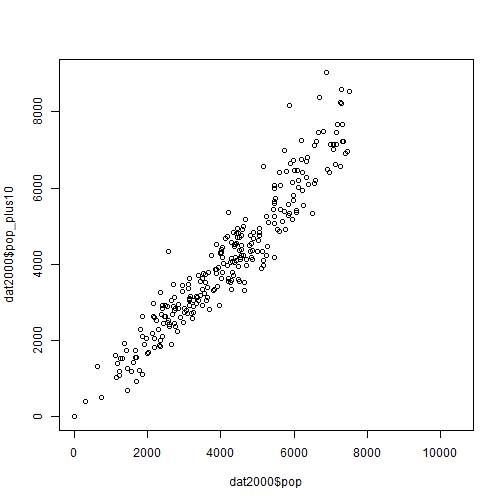The density and histogram functions are convenient ways to understand the distribution of the data (in this case the 2010 population in each Census Tract). The population is somewhat normally distributed and ranges from around zero to 10,000.

``````plot(density(dat2000\$pop_plus10, na.rm = T))
``````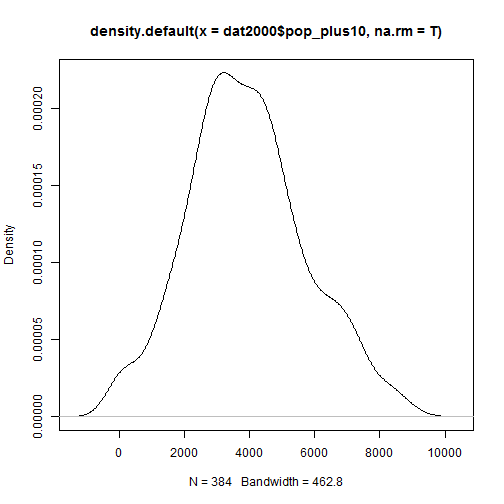``````hist(dat2000\$pop_plus10)
``````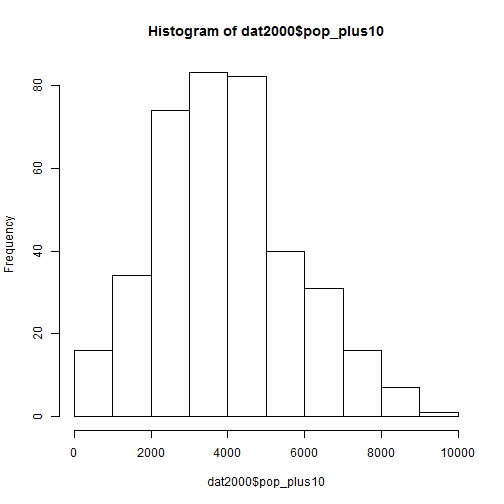Before proceeding, compare the Census Tracts that are consistent between 2000 and 2010 with those that are not. As you would expect, low population and high population Census Tracts were most likely to change boundaries.

``````hist(dat2000\$pop[is.na(dat2000\$pop_plus10)==T])
``````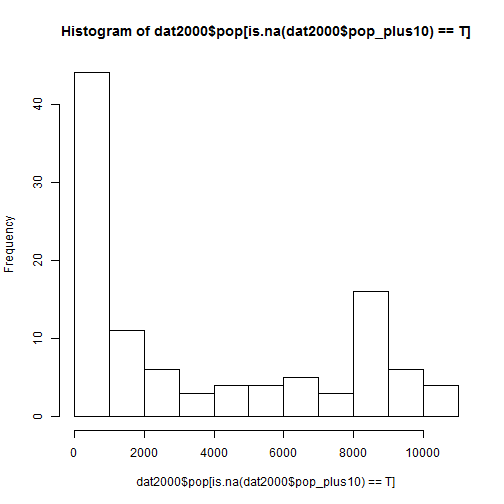``````hist(dat2000\$pop[is.na(dat2000\$pop_plus10)==F])
``````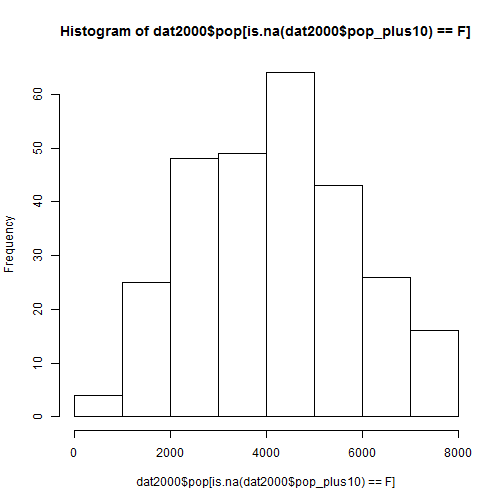How well can you predict the 2010 Census Tract population from the 2000 Census Tract population?

``````reg1 <- lm(pop_plus10~pop, dat2000)
summary(reg1)
``````
``````##
## Call:
## lm(formula = pop_plus10 ~ pop, data = dat2000)
##
## Residuals:
##      Min       1Q   Median       3Q      Max
## -1271.96  -357.73   -45.54   355.51  2334.68
##
## Coefficients:
##              Estimate Std. Error t value Pr(>|t|)
## (Intercept) -92.07364   88.72101  -1.038      0.3
## pop           1.01166    0.01986  50.938   <2e-16 ***
## ---
## Signif. codes:  0 '***' 0.001 '**' 0.01 '*' 0.05 '.' 0.1 ' ' 1
##
## Residual standard error: 552 on 273 degrees of freedom
##   (215 observations deleted due to missingness)
## Multiple R-squared:  0.9048, Adjusted R-squared:  0.9045
## F-statistic:  2595 on 1 and 273 DF,  p-value: < 2.2e-16
``````

The R-squared statistic indicates that the 2000 population explains about 90% of the variation in the 2000 population. The following shows how well the regression line fits the data.

``````plot(dat2000\$pop, dat2000\$pop_plus10)
abline(reg1)
``````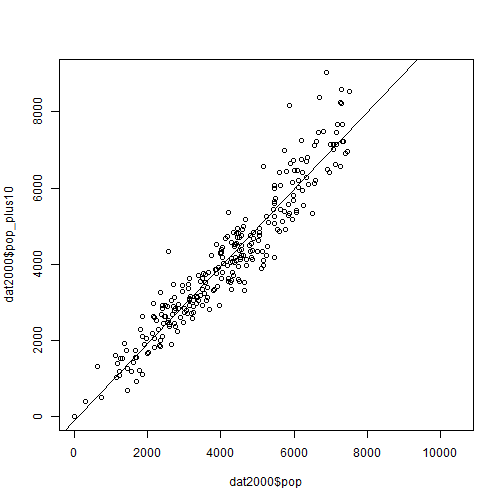Furthermore, each person in 2000 correlates with 1.01 person in 2010, though with a standard error of 0.02, this is hardly a ringing endorsement of population growth. Plotting the population change from 2000 to 2010 shows that almost the same number of Census Tracts gained and lost population.

``````plot(density(dat2000\$pop_change, na.rm = T))
``````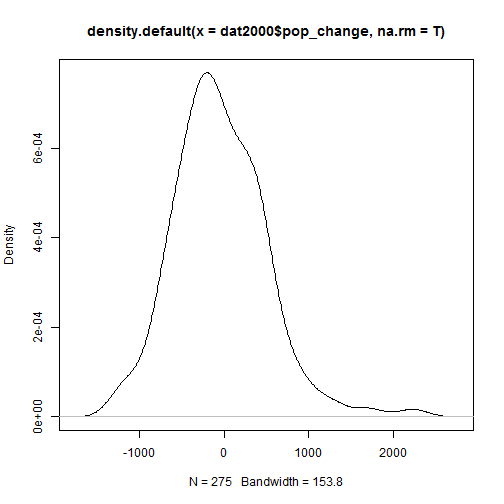Plotting the error term shows that the regression line fits the low population tracts a bit better than the high population tracts. (Note how closely this tracks with the population change number, since the prediction of the 2010 population relies entirely on the 2000 population.)

``````plot(density(resid(reg1)))
``````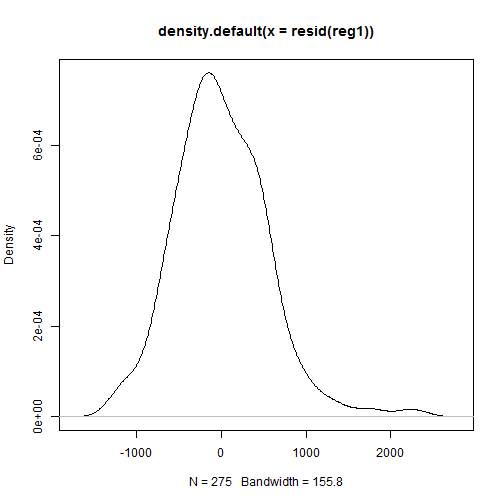Plotting the predicted population against the error terms reveals some additional patterns to help understand the regression. Ideally this pattern would look much more random with points equally and randomly distributed around the zero line.

``````plot(predict(reg1), resid(reg1))
abline(h=0,col=3,lty=3)
``````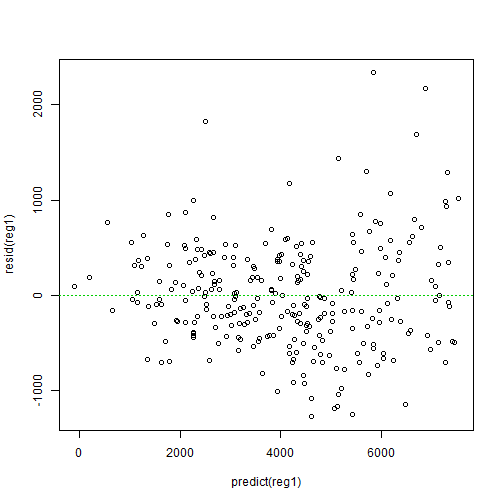One way to try to deal with under predicting the more populous Census Tracts is to introduce a quadratic term.

``````reg2 <- lm(pop_plus10~pop + I(pop^2), dat2000)
summary(reg2)
``````
``````##
## Call:
## lm(formula = pop_plus10 ~ pop + I(pop^2), data = dat2000)
##
## Residuals:
##      Min       1Q   Median       3Q      Max
## -1228.67  -338.37   -48.65   365.88  2334.17
##
## Coefficients:
##              Estimate Std. Error t value Pr(>|t|)
## (Intercept) 4.354e+02  1.750e+02   2.488 0.013460 *
## pop         7.114e-01  8.863e-02   8.027 3.02e-14 ***
## I(pop^2)    3.587e-05  1.033e-05   3.473 0.000599 ***
## ---
## Signif. codes:  0 '***' 0.001 '**' 0.01 '*' 0.05 '.' 0.1 ' ' 1
##
## Residual standard error: 541.2 on 272 degrees of freedom
##   (215 observations deleted due to missingness)
## Multiple R-squared:  0.9088, Adjusted R-squared:  0.9082
## F-statistic:  1356 on 2 and 272 DF,  p-value: < 2.2e-16
``````

Does this fit the data better? A full model vs. reduced model Anova test suggests that it does. So does the small improvement to the adjusted R-Squared.

``````anova(reg1,reg2)
``````
``````## Analysis of Variance Table
##
## Model 1: pop_plus10 ~ pop
## Model 2: pop_plus10 ~ pop + I(pop^2)
##   Res.Df      RSS Df Sum of Sq     F    Pr(>F)
## 1    273 83185653
## 2    272 79653918  1   3531735 12.06 0.0005991 ***
## ---
## Signif. codes:  0 '***' 0.001 '**' 0.01 '*' 0.05 '.' 0.1 ' ' 1
``````

The residual plot looks better…slightly…perhaps…

``````plot(predict(reg2), resid(reg2))
abline(h=0,col=3,lty=3)
``````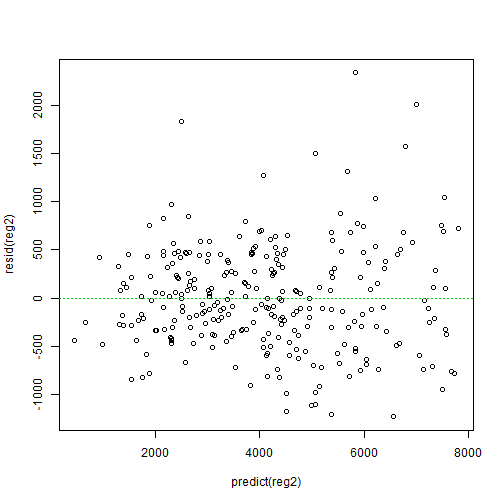Try testing some more interesting hypotheses. For example, do you expect higher income neighborhoods to grow more or less quickly than lower income neighborhoods? You could probably construct a reasonable theory in either direction, but my guess is that wealthier Census Tracts in Philadelphia were the most likely to grow.

``````reg3 <- lm(pop_plus10 ~ pop + I(pop^2)+ I(dat2000\$median_hh_inc/1000), dat2000)
summary(reg3)
``````
``````##
## Call:
## lm(formula = pop_plus10 ~ pop + I(pop^2) + I(dat2000\$median_hh_inc/1000),
##     data = dat2000)
##
## Residuals:
##      Min       1Q   Median       3Q      Max
## -1167.48  -361.12   -48.03   332.20  2313.85
##
## Coefficients:
##                                Estimate Std. Error t value Pr(>|t|)
## (Intercept)                   2.836e+02  1.959e+02   1.448 0.148838
## pop                           7.188e-01  8.843e-02   8.128 1.56e-14 ***
## I(pop^2)                      3.512e-05  1.030e-05   3.408 0.000753 ***
## I(dat2000\$median_hh_inc/1000) 4.375e+00  2.570e+00   1.702 0.089840 .
## ---
## Signif. codes:  0 '***' 0.001 '**' 0.01 '*' 0.05 '.' 0.1 ' ' 1
##
## Residual standard error: 539.3 on 271 degrees of freedom
##   (215 observations deleted due to missingness)
## Multiple R-squared:  0.9098, Adjusted R-squared:  0.9088
## F-statistic: 911.2 on 3 and 271 DF,  p-value: < 2.2e-16
``````

Every \$1,000 more in median income correlates with 4 more people in 2010, not a tremendously strong relationship. Furthermore, the coefficient is not statistically difference from 0 with 95% confidence (though it is with 90% confidence).

It also may be correlated with other variables that are associated with increasing or decreasing population, such as race.

``````reg4 <-  lm(pop_plus10~ pop + I(pop^2)+ I(median_hh_income/1000) + I(100*pop_black/pop), dat2000)
summary(reg4)
``````
``````##
## Call:
## lm(formula = pop_plus10 ~ pop + I(pop^2) + I(median_hh_income/1000) +
##     I(100 * pop_black/pop), data = dat2000)
##
## Residuals:
##      Min       1Q   Median       3Q      Max
## -1306.97  -327.63    -4.05   289.08  2213.82
##
## Coefficients:
##                            Estimate Std. Error t value Pr(>|t|)
## (Intercept)               6.204e+02  2.224e+02   2.789 0.005667 **
## pop                       7.208e-01  9.168e-02   7.862 9.19e-14 ***
## I(pop^2)                  3.365e-05  1.062e-05   3.170 0.001703 **
## I(median_hh_income/1000) -4.582e-01  2.871e+00  -0.160 0.873310
## I(100 * pop_black/pop)   -3.354e+00  9.524e-01  -3.521 0.000505 ***
## ---
## Signif. codes:  0 '***' 0.001 '**' 0.01 '*' 0.05 '.' 0.1 ' ' 1
##
## Residual standard error: 528.9 on 269 degrees of freedom
##   (216 observations deleted due to missingness)
## Multiple R-squared:  0.9122, Adjusted R-squared:  0.9109
## F-statistic: 698.6 on 4 and 269 DF,  p-value: < 2.2e-16
``````

Each percentage point higher concentration of black residents in Census Tracts in 2000 correlates with 3 fewer people in 2010. Using this regression, let's use the predict function to estimate Philadelphia's 2010 population:

``````sum(predict(reg4))
``````
``````##  1126700
``````

This is quite a bit lower than the actual 2010 population count:

``````sum(dat2000\$pop_plus10, na.rm=T)
``````
``````##  1504950
``````

The regression, however, excludes the hundred or so Census Tracts that changed between 2000 and 2010. Adding these back makes the prediction much closer to the actual population.

``````sum(predict(reg4, newdata = dat2000), na.rm =T)
``````
``````##  1543117
``````

Finally, use a similar command to estimate the 2020 population by applying our regression equation to the 2010 Census data.

``````sum(predict(reg4, newdata = dat2010), na.rm =T)
``````
``````##  1488331
``````

EXERCISE

1. Despite recent population growth, the model predicts that population will decline between 2010 and 2020. Do you agree with this prediction? What do you think is causing it?

2. Replace median income with a measure of the proportion of the population over 25 with a college degree of higher (edu_collegeplus), Do you expect neighborhoods with a higher proportion of well-educated residents to have grown or lost population between 2000 and 2010? Describe the results of the model in relationship to your expectations.

3. Does this change improve the model? Be sure to consider statistical fit, residual plots, and theory in providing your answer.

4. Develop a different set of models predicting population change (pop_change) instead of the population ten years later (pop_plus10). What factors do you expect to be good predictors of total population change? Describe the model, model fits, and residual plots.

This entry was posted in Planning Methods. Bookmark the permalink.